# Dimensional analysis question

• Jaydude
In summary, Jay attempted to solve the equation for 10 K_Q using the straight line method but was unable to do so. He is now looking for other methods to solve the equation.f

#### Jaydude

Hi, this is my first post, so sorry if this is posted on wrong section etc.

Do not worry about the initial part of dimensional analysis, that was fine - use Buckingham pi therem, but here's the prob...

Below is the question, I am stuck on being part C, however to show you guys what needs to be done, I've shown my working for parts a and b, and what I attempted for part C: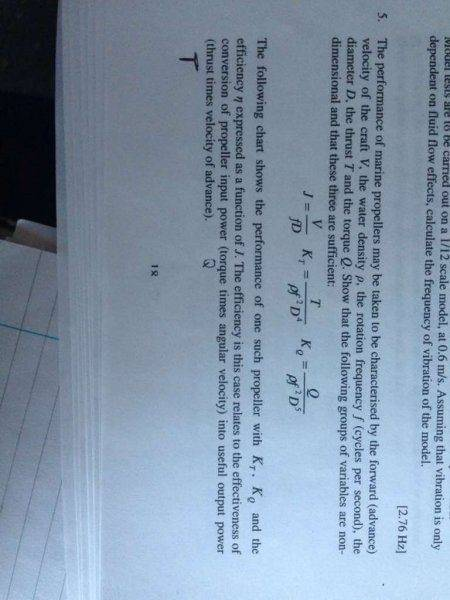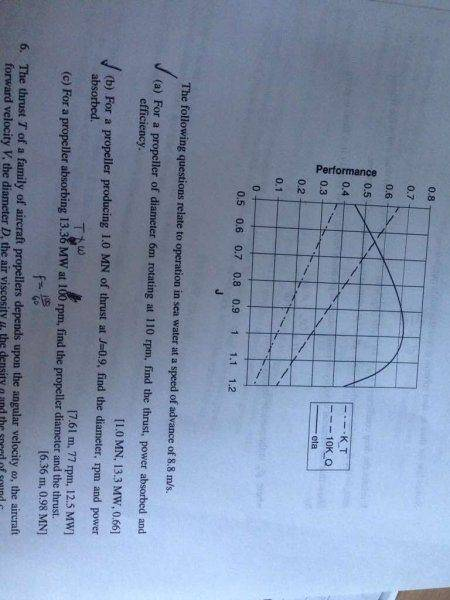My working: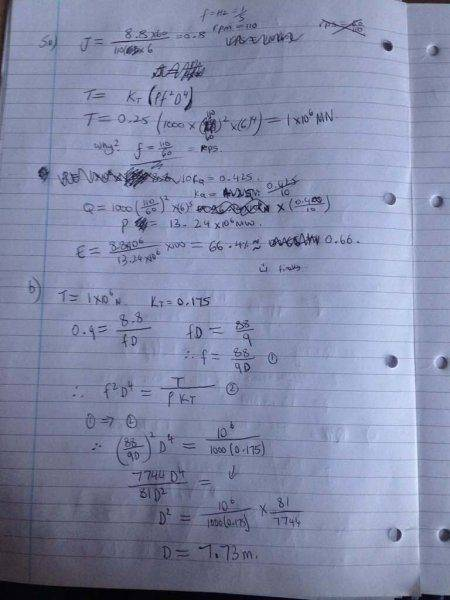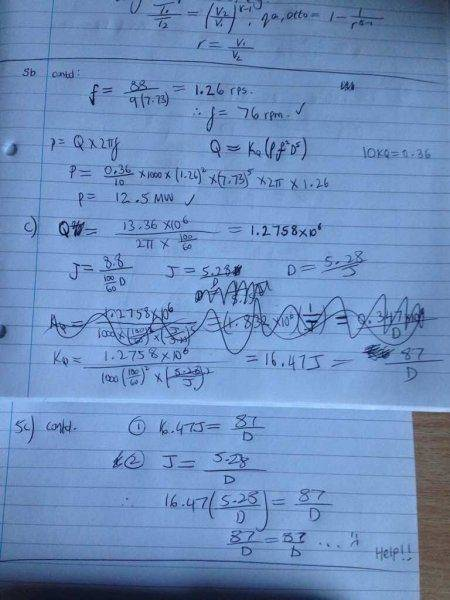Please help, I've been trying to work out q5 for hours, really need to move on! (Tutors do not provide solutions so you guys are my only hope)

Kind regards, Jay.

I suggest you rotate the images of the textbook so people don't have to read them sideways - or explain how they can be rotated with tools on the forum.

So, my neck is now kind of stiff, but I have a suggestion: It should be fairly easy to find an expression for, for instance, ##10 K_Q## in terms of ##J## (what is the equation of a straight line etc.).

You'd then have something like:
$$J = \frac{V}{f D}\\ 10 K_Q = a J + b = \frac{10 Q}{\rho f^2 D^5}$$to work with.

One problem I see is that you've done all your calculations assuming that ρ of seawater is 1000 kg/m3. It's actually about 1025 kg/m3.

I assumed my question got deleted due to a pm I got, only just found I got replies - thanks!

The straight line method seems like a good idea- something I dismissed thinking it was too much for this question, maybe not. I'll post further workings soon if I get stuck/solve it.

I assumed my question got deleted due to a pm I got, only just found I got replies - thanks!

The straight line method seems like a good idea- something I dismissed thinking it was too much for this question, maybe not. I'll post further workings soon if I get stuck/solve it.
If you post any more images with your work, take more care than you did the first time. As already noted, the images of the problem were posted sideways. Also, your work was pretty messy, with several sections scribbled over. If you want us to help, don't make us have to work too hard to do so.

I had another go, but the equation at the end is...near impossible to solve - pics below: (I'm being as neat, clear and concise as possible!)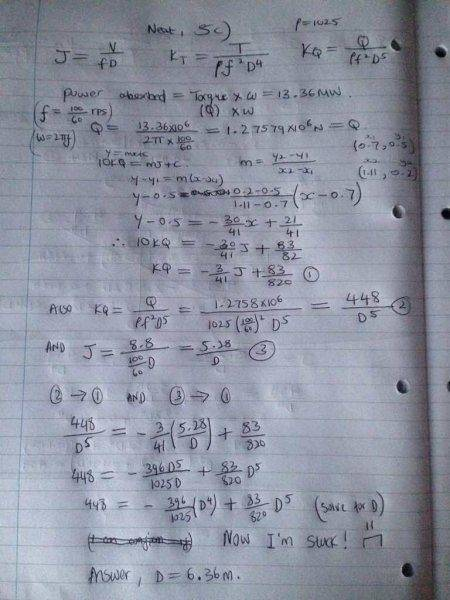Answer is 6.36m, if I enter that into the equation I get at the end, it's a close enough value, so the equation I think is right, just how would I solve that?!
Any other methods that can be recommended?

Thanks and kind regards - Jay

For polynomials of degree 5 or higher, you either solve them graphically by plotting them, or you use iteration. Since it's a polynomial in one variable (namely D), you can use Newton's method to speed up convergence on a solution.

http://en.wikipedia.org/wiki/Newton's_method